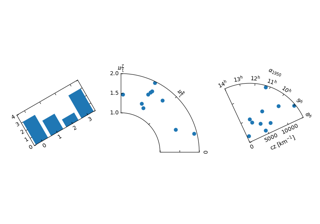# mpl_toolkits.axisartist.floating_axes.GridHelperCurveLinear¶

class mpl_toolkits.axisartist.floating_axes.GridHelperCurveLinear(aux_trans, extremes, grid_locator1=None, grid_locator2=None, tick_formatter1=None, tick_formatter2=None)[source]

aux_trans : a transform from the source (curved) coordinate to target (rectilinear) coordinate. An instance of MPL's Transform (inverse transform should be defined) or a tuple of two callable objects which defines the transform and its inverse. The callables need take two arguments of array of source coordinates and should return two target coordinates.

e.g., x2, y2 = trans(x1, y1)

__init__(self, aux_trans, extremes, grid_locator1=None, grid_locator2=None, tick_formatter1=None, tick_formatter2=None)[source]

aux_trans : a transform from the source (curved) coordinate to target (rectilinear) coordinate. An instance of MPL's Transform (inverse transform should be defined) or a tuple of two callable objects which defines the transform and its inverse. The callables need take two arguments of array of source coordinates and should return two target coordinates.

e.g., x2, y2 = trans(x1, y1)

__module__ = 'mpl_toolkits.axisartist.floating_axes'
get_boundary(self)[source]

Return (N, 2) array of (x, y) coordinate of the boundary.

get_data_boundary(self, side)[source]

Return v=0, nth=1.

get_gridlines(self, which='major', axis='both')[source]

Return list of grid lines as a list of paths (list of points).

which : "major" or "minor" axis : "both", "x" or "y"

new_fixed_axis(self, loc, nth_coord=None, axis_direction=None, offset=None, axes=None)[source]

## Examples using mpl_toolkits.axisartist.floating_axes.GridHelperCurveLinear¶